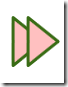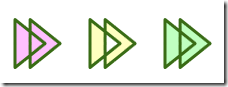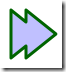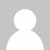I have been attempting to make some nice looking buttons to use with my Silverlight Audio Player, and came up with a basic design in XAML, borrowing some colour ideas from iTweek's icons on deviantart. The XAML for the basic play button with drop shadow is:

``````<Ellipse Canvas.Left="53" Canvas.Top="76" Width="26" Height="8" Fill="#40000000" />
<Ellipse Canvas.Left="50" Canvas.Top="50" Stroke="#396C15" Width="32" Height="32">
<Ellipse.Fill>
</Ellipse.Fill>
</Ellipse>
<Path Canvas.Left="50" Fill="#FFFFFF" Stroke="#396C15" Data="M 13,58 l 10,8 l -10,8 Z" StrokeLineJoin="Round" />
``````

Things got a bit more interesting when I tried to make a fast-forward icon by overlapping two triangles. Simply drawing two triangles over the top of each other does not give the desired result:

``````<Path Canvas.Left="80" Fill="#FFC0C0" Stroke="#396C15" Data="M 13,58 l 10,8 l -10,8 Z "
StrokeLineJoin="Round"  />
<Path Canvas.Left="80" Fill="#FFC0C0" Stroke="#396C15" Data="M 18,58 l 10,8 l -10,8 Z "
StrokeLineJoin="Round"  />
``````The `Path` `Data` property allows you to specify more than one closed shape, but this results in the strokes of both triangles being visible rather than combining to form one outline. This happens whether you use the `Path` syntax or create a `GeometryGroup` and add `PathGeometrys`. Rather annoyingly PathGeometry does not have a `Data` property, which makes for some cumbersome XAML:

``````<Path Canvas.Left="105" Fill="#FFC0FF" Stroke="#396C15" Data="F 1 M 13,58 l 10,8 l -10,8 Z M 18,58 l 10,8 l -10,8 Z"
StrokeLineJoin="Round"  />

<Path Canvas.Left="130" Fill="#FFFFC0" Stroke="#396C15" Data="M 13,58 l 10,8 l -10,8 Z M 18,58 l 10,8 l -10,8 Z"
StrokeLineJoin="Round"  />

<Path Canvas.Left="155" Fill="#C0FFC0" Stroke="#396C15" StrokeLineJoin="Round">
<Path.Data>
<GeometryGroup FillRule="NonZero">
<PathGeometry>
<PathFigure StartPoint="13,58" IsClosed="True">
<LineSegment Point="23,66"  />
<LineSegment Point="13,74"  />
</PathFigure>
</PathGeometry>
<PathGeometry>
<PathFigure StartPoint="18,58" IsClosed="True" >
<LineSegment Point="28,66"  />
<LineSegment Point="18,74"  />
</PathFigure>
</PathGeometry>
</GeometryGroup>
</Path.Data>
</Path>
``````This is still not the effect I want, irrespective of the `FillRule` used. The solution in WPF is to use a CombinedGeometry. This allows two `PathGeometrys` to be specified that can be combined as a union to create one shape. Again we have very verbose XAML because we can't use the `Path` mini language:

``````<Path Canvas.Left="180" Fill="#C0C0FF" Stroke="#396C15" StrokeLineJoin="Round">
<Path.Data>
<CombinedGeometry GeometryCombineMode="Union">
<CombinedGeometry.Geometry1>
<PathGeometry>
<PathFigure StartPoint="13,58" IsClosed="True">
<LineSegment Point="23,66"  />
<LineSegment Point="13,74"  />
</PathFigure>
</PathGeometry>
</CombinedGeometry.Geometry1>
<CombinedGeometry.Geometry2>
<PathGeometry>
<PathFigure StartPoint="18,58" IsClosed="True" >
<LineSegment Point="28,66"  />
<LineSegment Point="18,74"  />
</PathFigure>
</PathGeometry>
</CombinedGeometry.Geometry2>
</CombinedGeometry>
</Path.Data>
</Path>
``````

This produces the shape I wanted:But now we run into another problem. The Silverlight 2 beta does not support CombinedGeometry, and I have no idea if this is going to be supported as part of the full release of Silverlight 2.

So how can we get this in Silverlight? At the moment I know of only two solutions:

1. Do the maths yourself. For two triangles as in my example, this wouldn't be too hard, but it would be a real pain if your combined shape involved any curves or ellipses.

2. Get Expression Blend to do it for you. Draw the two shapes, select them, then select Object | Combine | Unite. This will create a single path you can use in a Silverlight application. It doesn't leave the XAML quite how I would like it (adding margins and using absolute coordinates in the path, rather than relative).

``````<Path Height="17"
HorizontalAlignment="Left"
Margin="138.5,91.5,0,0"
VerticalAlignment="Top"
Width="16" Fill="#C0C0FF" Stretch="Fill"
Stroke="DarkGreen"
StrokeLineJoin="Round"
Data="M0.5,0.5 L5.5,4.5 L5.5,0.5 L15.5,8.5 L5.5,16.5 L5.5,12.5 L0.5,16.5 L0.5,0.5 z"/>
``````Good article dude!

Anonymouscan you suggest how to achieve something like this shown in this website?

http://www.web-demographics.com:8085/

I want to have similar menu bar (both horizantal and vertical) which I want to hide when mouse is away but not the ellipse.

AnonymousThanks for this tip !
If you have the first path filled in red, and the second in yellow, then the combined path is filled in yellow... the red color is lost !
Do you have an idea pleaese ?